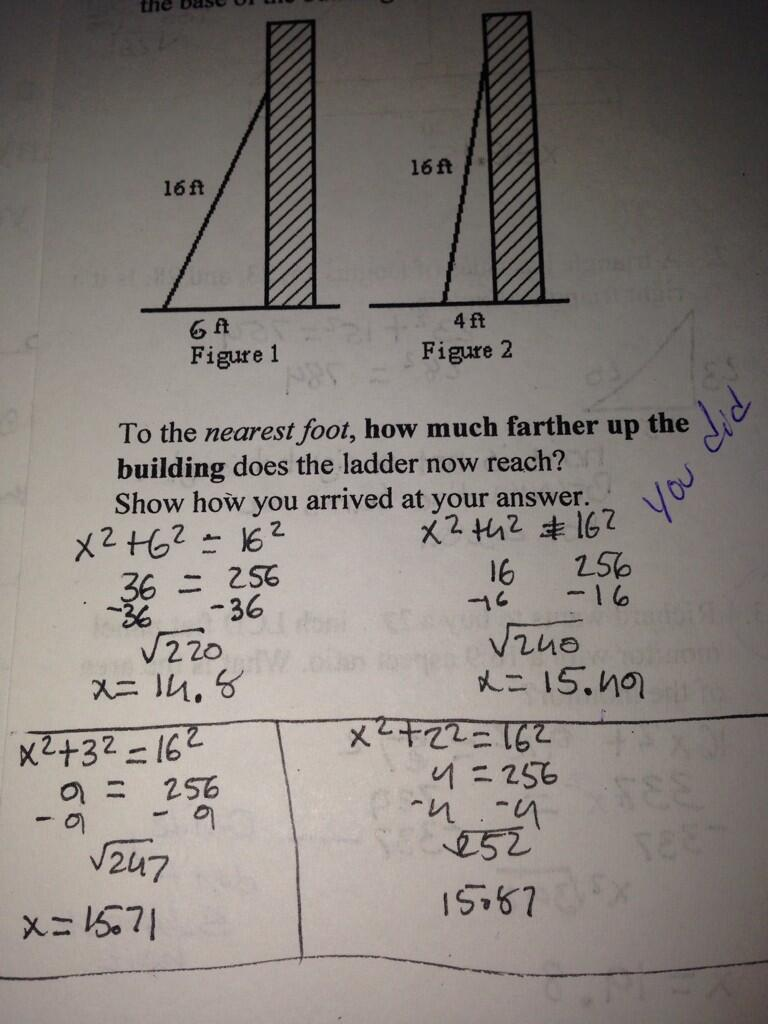CategoriesLet’s help Lisa out in the comments, mmk?

## 4 replies on “Pythagorean Theorem and Ladders”Θανάσηςsays:

I don’t understand where the misunderstanding or problem is. Are you referring to the fact that the student did not answer the *exact* question (on the difference of height)? Otherwise all info is there, and the approach seems valid and correct to me.
Thanassis

I think the question is why they continued with the 3^2 and 2^2 and got more numbers instead of subtracting the two they had. What do these two values of x mean at the bottom?

I’d be helping the student write correct equations (and not 16 = 256) though of course we know what they mean. And labeling diagrams so we know what x refers to in each case. But the additional two paragraphs at the bottom completely mystify me.

I think the student didn’t fully understand what the question was asking for, saw that sqrt(240) was not 1 larger than sqrt(220) and just kept going.Laura C.says:

I’ve found that a lot of the time, asking students to do 2 different things sequentially in the same problem confuses them. I had a student ask me for help with the following problem in a remedial math class:

One \$10 bag of fertilizer will cover 310 square feet. Approximately what would it cost to cover 2200 square feet?

The problem was in the “Division” section, so the student knew that there had to be division done–but the book made an error that tripped zir up. Instead of rounding up to 8 bags, to ensure that there would be no uncovered patches left, the book rounded down to 7. So clearly, the student’s answer of \$80 was wrong, and there must be some obscure formula that the teacher knows, that will make it work out right.

I’ve also had students work problems in which a person deposits money into an account, then writes checks. But even right after I explain that writing checks takes money out of a bank account, the students will add up all of the numbers–because why would there be 2 different operations?

Or worse, when the problem says, “4 different plays had an attendance of A, B, C, and D respectively,” to find the total number of attendees, the students will add A, B, C, and D, and then multiply by 4, because other problems required 2 operations, so clearly this one will, too.

I think it may be a mental block of the “math isn’t applicable to real life; it’s just a set of rules” mindset, but I’m not sure. Talking to them about “distractor numbers” helps with some of these issues, but not all.